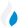•Trade Pi
•Trade
•Exchange
•Trade Pi
•Trade
•Exchange
\$1.1T
Total marketcap
\$65.86B
Total volume
39.99%
BTC dominance
•Trade Pi
•Trade
•Exchange

# trix to KRW Exchange Rate - 1 TriumphX in KRW

0.5113
Vol [24h]
\$34,162.45

## trix to KRW converter

Exchange Pair Price 24h volume
Coinone TRIX/KRW \$0.0004063 \$34.01K

## TRIX/KRW Exchange Rate Overview

Name Ticker Price % 24h 24h high 24h low 24h volume
TriumphX trix \$0.0004301 0.3074% \$0.000431 \$0.0004122 \$50.04K

Selling 1 TriumphX trix you get 0.5113 KRW.

TriumphX Nov 18, 2020 had the highest price, at that time trading at its all-time high of \$36.35.

810 days have passed since then, and now the price is 0.00% of the maximum.

Based on the table data, the TRIX vs KRW exchange volume is \$34,162.45.

Using the calculator/converter on this page, you can make the necessary calculations with a pair of TriumphX to KRW.

## Q&A

### What is the current TRIX to KRW exchange rate?

Right now, the TRIX/KRW exchange rate is 0.5113.

### What has been the TriumphX to KRW trading volume in the last 24 hours?

Relying on the table data, the TriumphX to KRW exchange volume is \$34,162.45.

### How can I calculate the amount of KRW? / How do I convert my TriumphX to KRW?

You can calculate/convert KRW from TRIX to KRW converter. Also, you can select other currencies from the drop-down list.

## TRIX to KRW Сonversion Table

TRIX KRW
2000 TRIX = 1,022.6 KRW
3000 TRIX = 1,533.9 KRW
5000 TRIX = 2,556.5 KRW
8000 TRIX = 4,090.4 KRW
20000 TRIX = 10,226 KRW
30000 TRIX = 15,339 KRW
40000 TRIX = 20,452 KRW
200000 TRIX = 102,260 KRW
300000 TRIX = 153,390 KRW
3000000 TRIX = 1,533,900 KRW
30000000 TRIX = 15,339,000 KRW
300000000 TRIX = 153,390,000 KRW
3000000000 TRIX = 1,533,900,000 KRW